# Self Supervised Learning in Audio and Speech

Learning speech representations from raw audio in an unsupervised training fashion. Made by Tulasi Ram Laghumavarapu using Weights & Biases
Tulasi Ram Laghumavarapu

This report will discuss unsupervised pre-training of unlabelled audio data and use the pre-trained model for downstream tasks such as speech recognition and speaker identity from raw audio. Specifically, we will be discussing variants of wav2vec and discuss how to build speech recognition systems with low resource languages.

If you can build a decent speech recognition system with just 10min of labeled data? Interesting, isn’t it? To know more, please go ahead.

## Introduction

Speech Recognition systems are data-hungry. They need 100s of hours of labeled data to achieve a decent Word Error Rate(WER). However, the availability of labeled data in low-resource languages is significantly less. Data annotation is expensive both in terms of resources and time. In India alone, there are 122 major languages and 1599 other languages. Building speech recognition systems in low-resource languages are not feasible.

Wav2Vec outperformed DeepSpeech2 while using two orders of lesser magnitude using a pre-trained model trained on unlabelled data trained on the LibriSpeech dataset.

Unsupervised learning is quite common in NLP and Computer Vision. In NLP models like BERT, GPT, ULMFit employ this technique, while in Computer Vision models like SimCLR, Swav use it.

## Research Work

Some of the unsupervised techniques in the speech domain are:

In this blog post, we will be focusing mainly on multiple variants of Wav2Vec along with references from other papers whenever necessary.

## Wav2Vec

Wav2Vec is an unsupervised pre-training approach for speech recognition by learning representations of raw audio. In general, we use log mel filter banks or mfcc features to train speech recognition systems. Instead, if we use the encoding or representation of Wav2Vec we can achieve almost similar results while using two orders of magnitude less labeled training data.

### Data Used For Pre-training:

1. Wall Street Journal (WSJ): 81hrs of transcribed data
2. Libri Speech: 960hr full training-data
3. Libri Speech: 80hr subset of cleaned data

### Model

The Wav2Vec model is Fully Convolutional. It consists of two networks:

1. Encoder Network
2. Context Network

Before going further, let us get familiarized with the term Latent Space.

** Latent Space**: In simpler words, it is the space where two representations of the vectors will be closer if they are similar. For example, in the case of word embeddings, words with similar meanings will be close in the latent space representation. Similarly, in audio, waveforms with similar characteristics like prosody, pitch, speaker identity, etc. will be close in latent space.

Encoder Network: It takes raw audio(X) as input and embeds the audio signal into latent space representation(Z). It is a five-layer full convolutional network with kernel sizes [10, 8, 4, 4, 4] and strides [5, 4, 2, 2, 2]. Also, there is a larger version of wav2vec with 2 more CNN layers and used in larger training datasets(wav2vec large).

The encoder encodes about 30ms of 16kHz audio with striding of 10ms and generates low frequency representation(Z). This latent space representation will then be fed into Context Network.

The existence of Encoder Network because of these two reasons:

1. Encoder generates low dimensional compact latent space representation, which can be handled by the context network easily for predicting the future.
2. I also believe one more reason is instead of predicting the future on raw audio data which varies very fastly(like noise, details) w.r.t time we use Encoder Network representations which contains some meaningful data to predict the future.

Context Network: The latent space representations(Z) are further encoded by a bigger stack of 9 convolutional layers and also mixes with multiple latent representations to produce a contextualized representation(C) with a receptive field size of 210ms.

$ci = g(zi . . . zi−v)$

Here g represents the context network, and v represents the receptive field.

Here is how the wav2vec model looks like: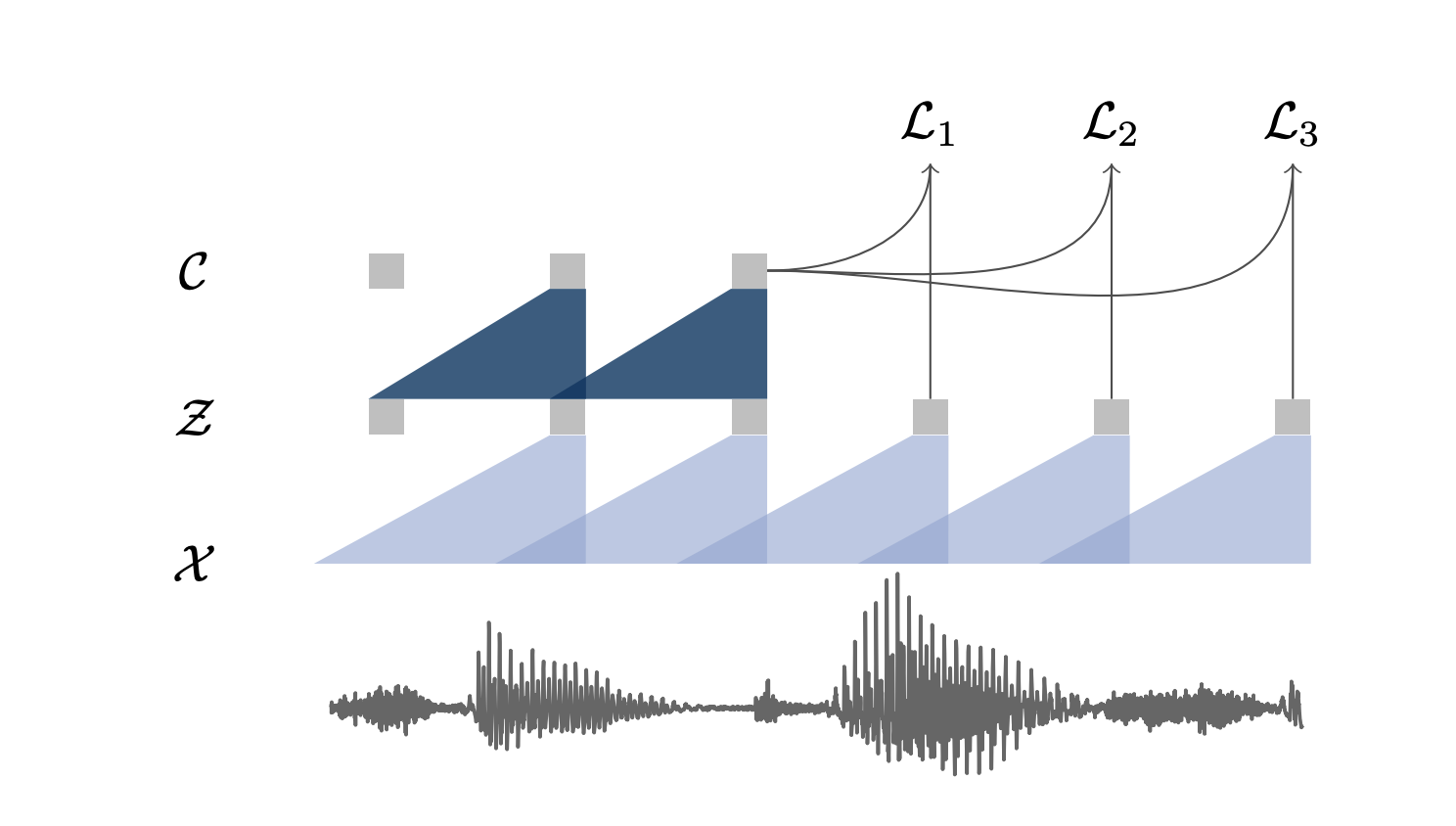Source: Original Wav2Vec Paper

All the layers in both the networks contain 512 channels, a group normalization layer with ReLU non-linearity.

This model architecture is similar to CPC(Contrastive Predictive Coding), except it is fully convolutional while the CPC is based on the GRU module.

### Training Objective:

These contextualized representations are then used to predict the future by contrasting the two latent speech representations from a set of negative samples.

We randomly select negative samples by uniformly choosing distractors from each audio sequence to predict if the given sample is in the near future from the current offset position. λ is the number of negative samples (10 leads to better performance according to the paper).

How do we select negative samples?

1. Sampling randomly from the entire dataset and encode them, which is not efficient.
2. Sampling randomly from the same minibatch.
• Sampling from the same sequence farther away from the target
• Sampling from the other examples of mini-batch.

Of course, we can use a mix of both from the 2nd point.

Which one to choose and when?

1. Suppose we are drawing the negative samples from other examples of mini-batch. The dataset is a multi-speaker dataset then negative samples most likely to be of different speakers. The model will try to learn the representations of speaker identity.
2. If we draw the negative samples from the same sequence, then the model will try to learn the representations that will capture phonetic information, prosody, etc. Models trained with this objective can be used for speech recognition downstream tasks.

### Loss:

The model distinguishes sample $z_{i+k}$( k time steps away) from negative samples drawn from proposal distribution($p_{n}$) by minimizing the contrastive loss for each step k.

$L_{k} = − \sum_{i=1}^{T-k}( log σ(z { i+k}^{T}h{k}(c_{i})) + λ {{z}^{~}\sim p{n}}E [log σ(−z^{T}h_{k}(c_{i}))])$

Here $c_{i}$ represents context vector representation at $i_{th}$ timestep. E represents expectation. $h_{k}$ represents step-specific affine transformation. It is applied to $c_{i}$ at each k.

$h_{k}(c_{i}) = W_{k}c_{i}+b_{k}$

$σ$ represents sigmoid function. $σ(z { i+k}^{T}h{k}(c_{i}))$ represents probability of $z_{i+k}$ being a true sample.

$E [log σ(−z^{T}h_{k}(c_{i}))]$ represents expectation of probability of negative samples(distractors) sampled from different sequences and speakers with a probability $p_{n}$ .

Finally, the loss is summed over all the k steps.

$L = \sum_{k=1}^{K}L_{k}$

Just to be clear wav2vec is not an acoustic model. Now once training is completed we feed the raw audio as input to the wav2vec and extract the contextualized representation. Extracted contextualized representations can be used as input to acoustic models like DeepSpeech2, Wav2Letter etc instead of log mel filter banks or mfcc features…

### Results:

The author’s used wav2letter as an acoustic model.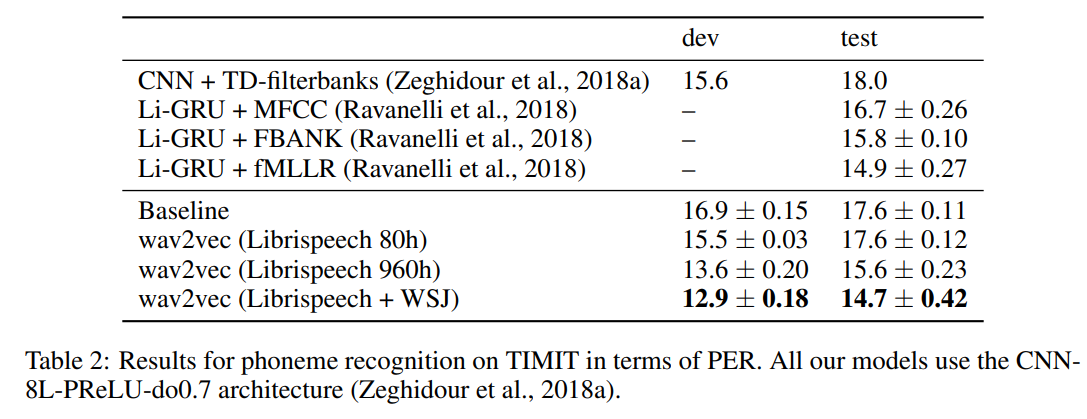It is clear from the results that pre-training on more data leads to better WER results. It is also evident that pre-trained representations are performing better than log-mel filter bank features. Pre-training reduces WER by 36% on the nov92 dev test when only 8hrs of labeled data is available.

This paper triggered more research in this field

### VQ-Wav2Vec:

Language has a structure and semantic unions that represents the context. In order to extract the information of basic units of speech, vq-wav2vec introduced a quantization module.

Discrete Latent Speech Representations: Discretization enables us to directly use well-performing NLP architectures like BERT to speech to learn more about language. Discretization of Speech features is achieved by introducing a quantization module in the wav2vec architecture.

### Model:

Model architecture is the same as wav2vec architecture, but also, a new quantization module is introduced to generate discrete features from dense feature representations. Here is the full architecture: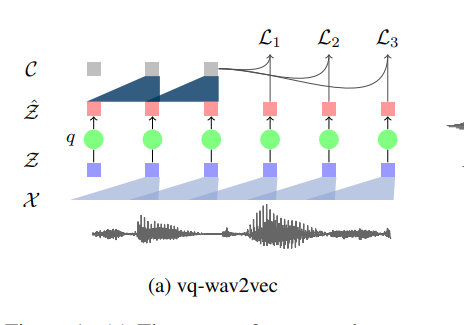This quantization module takes feature representation(Z) from the encoder and produces discrete representation(Z1) from a fixed-size codebook containing V representations of sizes. vq-wav2vec uses two methods to achieve this:

1. Gumbel-Softmax Quantization
2. Online K-Means: Vector from the code-book, which is closest in Euclidean space through dense representation(Z).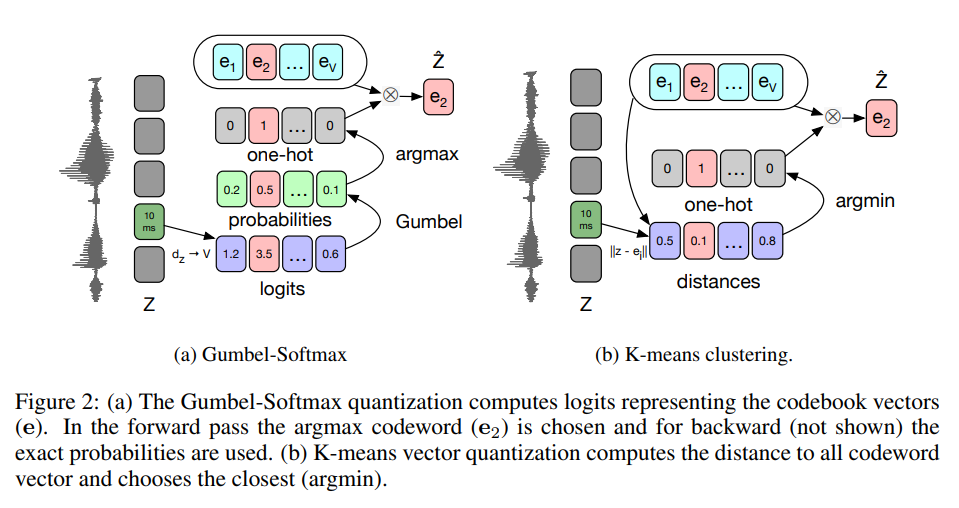To know more about these two quantization techniques, please refer to the paper.

Training Objective and Loss are the same as discussed from the original Wav2Vec paper.

Once training is completed, we will get the discretized representation of raw audio to feed to NLP architecture like BERT. To know more about BERT, please refer to these blogs by Jay Alammar

The training objective of BERT is to predict masked input tokens based on the surrounding context. Once the BERT model is trained, we can use the representations of BERT to feed into the acoustic model directly.

Below is the combined pipeline of Vq-wav2vec, BERT, and an acoustic model.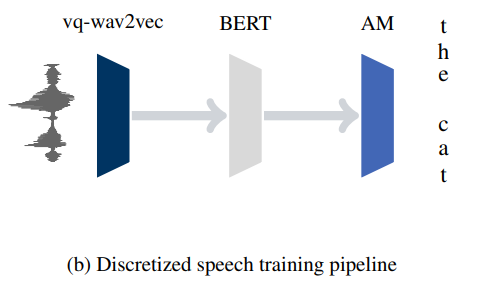### Results:

Results show that introducing the BERT pre-trained model achieved the state of art results on TIMIT phoneme classification data and the Wall Street Journal(WSJ). Let us look at the results: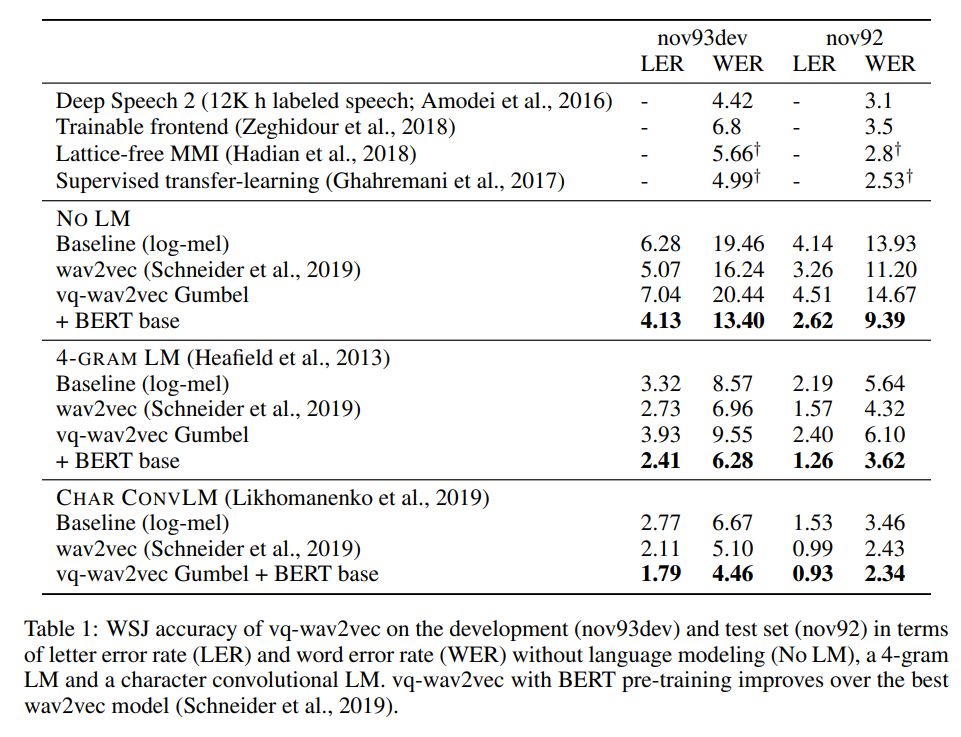The above results indicate that vq-wav2vec with Char ConvLM outperforms all other algorithms, including wav2vec with WER of 2.34 on the nov92 dataset.

Similarly for TIMIT dataset: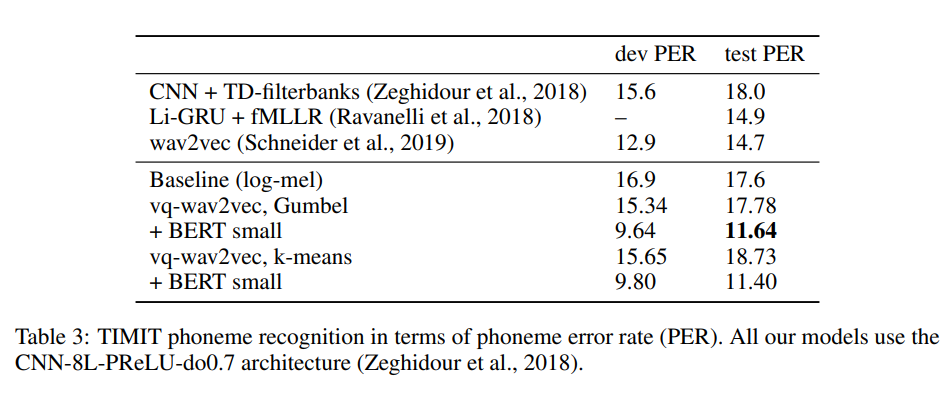PER: Phone Error Rate

One more exciting outcome of this paper is reducing bit-rate without affecting model performance(with the quantization module's help).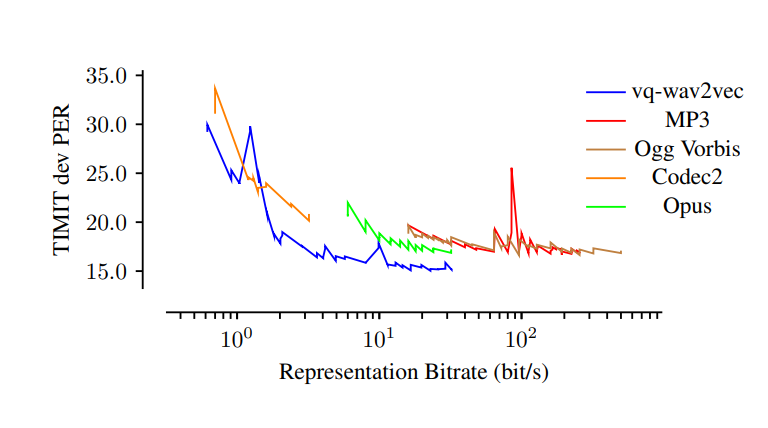Results showed that acoustic models on vq-wav2vec achieved the best results with all the available bit-rate settings.

## Wav2Vec2.0:

Wav2Vec2.0 is an improvised version of vq-wav2vec instead contextualized representations are learnt over continuous speech representation and self-attention captures dependencies over the sequence.

It is an end-to-end model that jointly learns discretized latent representations and contextualized speech representations from raw audio.

This paper proved that with just 10min of labelled data we can build a decent speech recognition system.

With just 10min of labelled data from LibriSpeech wav2vec2.0 achieved 4.8WER on LibriSpeech test-clean data which is a great deal.

### Model:

1. Model takes raw audio(X) as input and encodes into latent speech representations with the same stack of convolutional layers as wav2vec except ReLU activations are replaced by GeLU.
2. These latent space representations are masked(similar to what we do in BERT) then feeded into a transformer network(replaced aggregator network) to build contextual representations.
3. These latent speech representations are also fed into a quantization module that relies on product quantization. The choice of discretized latent speech representations led good results in vq-wav2vec paper.

Feature Encoder is the same as the one we discussed with wav2vec with minor changes.

Contextualized Representations with Transformer:

Aggregator network from wav2vec and vq-wav2vec replaced by Transformer network in wav2vec2.0. Instead of using fixed positional embeddings which encode absolute positioning information, author’s used a convolutional layer which acts as relative positional embedding.

Quantization Module:

Gumbel softmax version is used as a quantized module in this paper. Product quantization is used as a quantization strategy. It selects multiple quantized representations from G codebooks and concatenates them. Below is the image of model: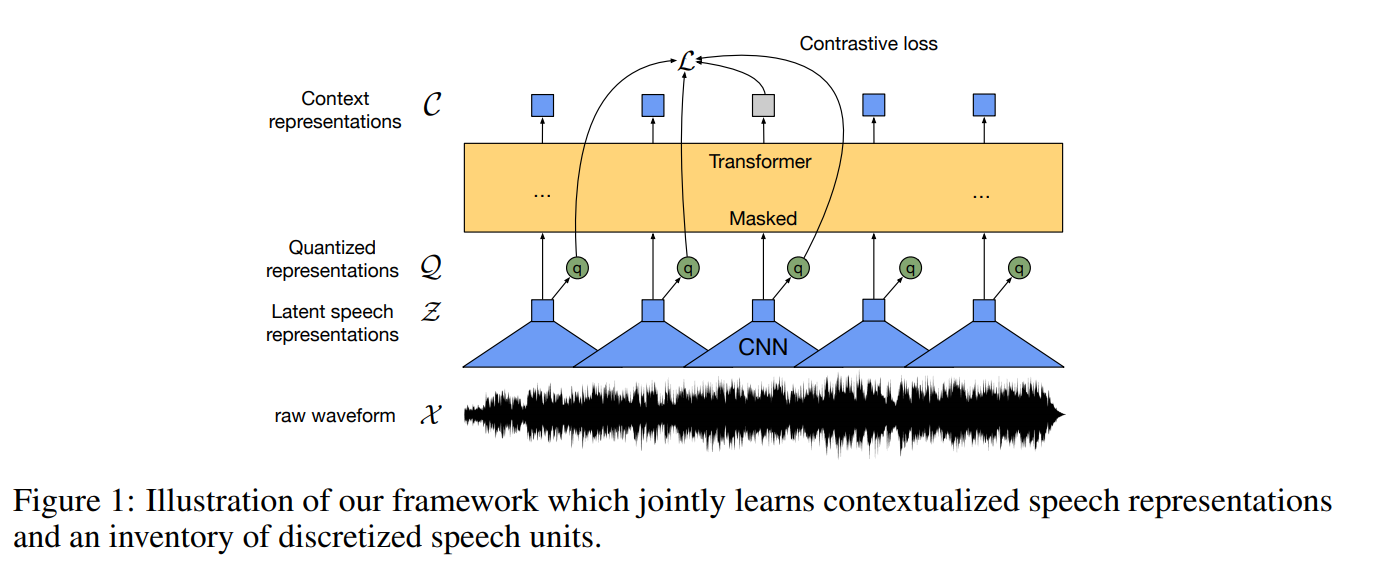1. Sample random starting points(p) without replacement from the input sequence. Author’s used 6.5percent as probability to select a starting point. It means each starting point as 6.5percent of chance to be selected as a starting point.

2. Mask M time steps(M=10 used in paper) from each sampled point. These spans can overlap as we randomly sampled starting points.

For example with p=2 and M=5 this is how figure looks: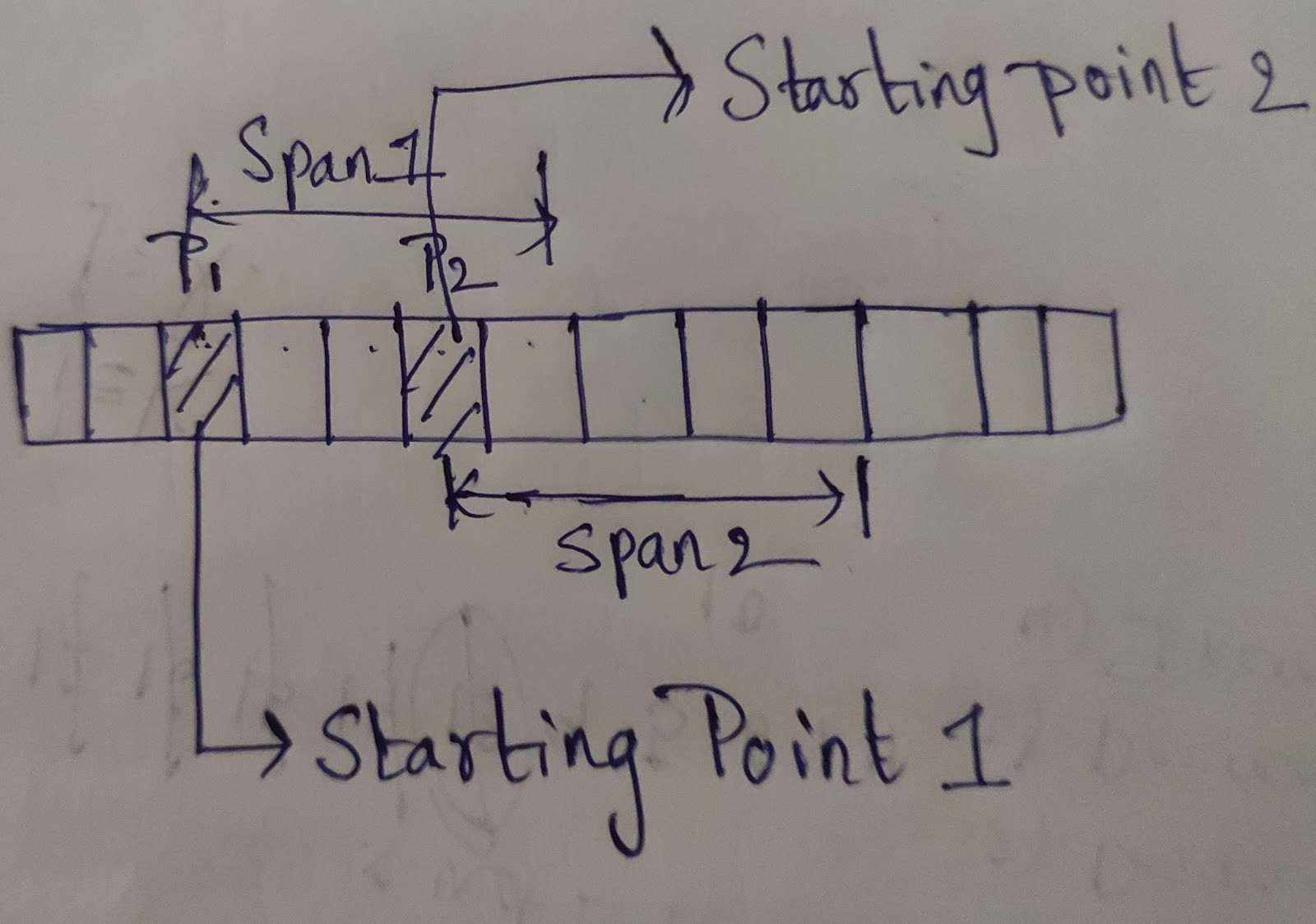### Training Objective:

Pre-training objective is to learn representations of raw audio by solving a contrasting task $L_{m}$ which requires to identify the true quantized latent speech representation for a masked time within a set of distractors. This is augmented by a code book diversity loss $L_{d}$ to encourage the model to use codebook entries quite often (from the paper)

$L = L_{m} + αL_{d}$

Contrastive Loss($L_{m}$) itself requires another blog post. To know more about Contrastive Loss refer to this blog post

Diversity Loss($L_{d}$) is designed to increase the use of quantized codebook representations(from quantization module) whereas $α$ is a hyper parameter.

Pre-training: Author’s experimented with two model configurations with same encoder setup but varying Transformer architectures Base and Large

Fine-tuning:

1. Once the model is pre-trained on unlabelled data in self-supervised fashion we will add a linear layer and initialize with random weights on top of the transformer network and retrain with few hrs of labelled data with CTC Loss.
2. Language Model is also used along with CTC. Language Models used in the paper.
• 4-gram Language Model
• Transformer LM with 16 attention heads(trained on LibriSpeech Corpus)
1. Encoder weights can be freezed while fine-tuning.

### Results:It is clear from the results that achieving 4.8WER with just 10min of labeled data is actually a big leap in self-supervised learning research.

It is also evident that if we increase the amount of labeling data we can get even better results.## Conclusion

Thank you for reading the article and I hope you got some insights to build a Speech Recognition system with fewer hrs of data.

I would like to thank Krisha Mehta for reviewing the article. Feel free to let me know the feedback through my Twitter account @Tulasi123789.

If you have any queries feel free to mail me at tulasiram11729@gmail.com